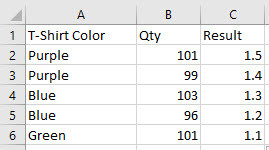# Multiple Nested AND / IF Statements

Do you have a spreadsheet that you want to label certain criteria if they have two conditions? In order to do this you can combine multiple IF with AND statements in Excel.

Below I have an example for a T-Shirt company that has an overabundance of certain t-shirts.We want to apply discounts to orders that contain blue and purple quantities;

• For any purple t-shirt orders that have a quantity equal to or over 100, a 1.5% discount will be applied.

• For any purple t-shirt orders under 100, a 1.4% discount will be applied.

• For any blue t-shirt orders equal to or over 100, a 1.3% discount will be applied.

• For any blue t-shirt orders under 100, a 1.2% discount will be applied.

• All other orders will receive a 1.1% discount.

For the above spreadsheet we want to create a nested AND/IF formula to apply the criteria to cell C2.

The formula will be as follows;

=

IF(AND(A2="purple",B2>=100),1.5,

IF(AND(A2="purple",B2<100),1.4,

IF(AND(A2="blue",B2>=100),1.3,

IF(AND(A2="blue",B2<100),1.2,

1.1))))

The breakout of this formula is as follows;

IF(AND(A2="purple",B2>=100),1.5,

If cell A2 contains the word "purple" and B2 is greater or equal to 100, enter a 1.5 discount.

IF(AND(A2="purple",B2<100),1.4,

If cell A2 contains the word "purple" and B2 is less than 100, enter a 1.4 discount.

IF(AND(A2="blue",B2>=100),1.3,

If cell A2 contains the word "blue" and B2 is greater or equal to 100, enter a 1.3 discount.

IF(AND(A2="blue",B2<100),1.2,

If cell A2 contains the word "blue" and B2 is less than 100, enter a 1.2 discount.

1.1))))

For all other orders, enter a 1.1 discount.

Copy and paste this formula into cell C2. Then paste it down to the other cells in the C column.

Your results will appear as below;